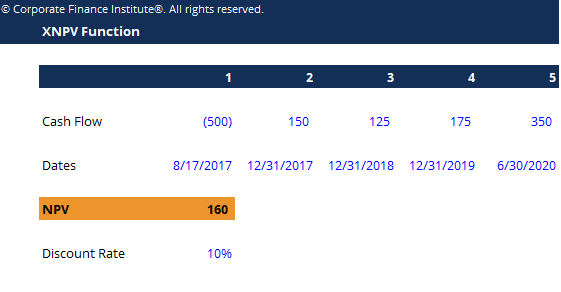# Excel for Finance Functions

## Excel for Finance Functions

This Excel for finance functions template demonstrates sample calculations using the top 10 most important functions and formulas for finance professionals.

Below is a preview of the XNPV function example:### Excel for Finance Functions Template

#### #1 XNPVXNPV Function in ExcelThe XNPV function in Excel should be used over the regular NPV function in financial modeling and valuation analysis to ensure precision and accuracy. The XNPV formula uses specific dates that correspond to each cash flow being discounted in the series. Learn step by step in this guide with examples and sceenshots

Determines the Net Present Value (NPV) of a series of cash flows at specific dates.

Formula: =XNPV(discount_rate, cash_flows, dates)

#### #2 XIRRXIRR FunctionThe XIRR function is categorized under Excel Financial functions. The function will calculate the Internal Rate of Return (IRR) for a series of cash flows that may not be periodic. If the cash flows are periodic, we should use IRR Function. In financial modeling, the XIRR function is useful in The XIRR function is

Determines the internal rate of return for a series of cash flows, given specific dates.

Formula: =XIRR(cash flows, dates)

#### #3 MIRRMIRR GuideThe Modified Internal Rate of Return (MIRR) is a function in Excel that takes into account the financing cost (cost of capital) and a reinvestment rate for cash flows from a project or company over the investment’s time horizon.

Determines the internal rate of return when the cash from one investment is invested in a different one.

Formula: =MIRR(cash flows, cost of borrowing, reinvestment rate)

#### #4 PMTPMT FunctionThe PMT function is categorized under Excel financial functions. The function helps calculate the total payment (principal and interest) required to settle a loan or an investment with a fixed interest rate over a specific time period.

Calculates the amount of payments given an interest rate, and a number of time periods and the total value of the loan.

Formula: =PMT(rate, number of periods, present value)

#### #5 IPMTIPMT FunctionThe IPMT function is categorized under Excel Financial functions. The function calculates the interest portion based on a given loan payment and payment period. We can calculate, using IPMT, the interest amount of a payment for the first period, last period, or any period in between.

Calculates the interest portion of a fixed debt payment.

Formula: = IPMT(rate, current period #, total # of periods, present value)

#### #6 EFFECT

Returns the effective annual interest rate for non-annual compounding.

Formula: =EFFECT(interest rate, # of periods per year)

#### #7 DB

Calculates the depreciation expense in each period.

Formula: =DB(cost, salvage value, life/# of periods, current period)

#### #8 RATERATE FunctionThe RATE function is categorized under Excel Financial functions. The function will calculate the interest rate charged on a loan or the rate of return needed to reach a specified amount on an investment over a given period.

Calculates the yield to maturity for a security.

Formula: =RATE(# of periods, coupon payment per period, price of bond, face value of bond, type)

#### #9 FVFV Function ExcelThe FV Function is categorized under Excel Financial functions. This function helps calculate the future value of an investment made by a business, assuming periodic, constant payments with a constant interest rate. Download the FV Function Excel file in this

Determines how much money you will have in the future, given a starting balance, regular payments, and a compounding interest rate.

Formula: =FV(rate, # of periods, payments, starting value, type)

#### #10 SLOPESLOPE FunctionThe SLOPE Function is categorized under Excel Statistical functions. It will return the slope of the linear regression line through the data points in known_y's and known_x's. In financial analysis, SLOPE can be useful in calculating beta for a stock. Formula = LOPE(known_y's, known_x's) The function uses the

Calculates Beta of a stock, given the weekly returns for the stock and the index you wish to compare it to.

Formula: =SLOPE(dependent variable, independent variable)

### More Free Templates

For more resources, check out our business templates library to download numerous free Excel modeling, PowerPoint presentation and Word document templates.

• Excel Modeling Templates
• PowerPoint Presentation Templates
• Transaction Document Templates

### Financial Analyst Certification

Become a certified Financial Modeling and Valuation Analyst (FMVA)® by completing CFI’s online financial modeling classes and training program!